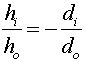Magnification

The size of the image relative to the object is given by the same formula as in the mirror case.A negative image height refers to an inverted image. Thus the general rule, Real images are inverted and virtual images are upright is true for both lenses and mirrors.

(This rule is not necessarily true if more than two lenses are involved. With two lenses, the object for the second lens is the image created by the first lens, and it can be behind the second lens. The usual formula 1/di + 1/do = 1/f still works in that case even though do is negative. But this situation will not be dealt with further in Physics 232.)

Lenses index      examples        Lecture index